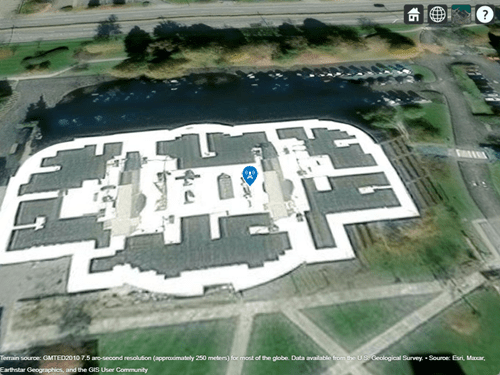# rxsite

## Description

Use the `rxsite` object to create a radio frequency receiver site.

## Creation

### Syntax

``rx = rxsite``
``rx = rxsite(coordsys)``
``rx = rxsite(Name,Value)``

### Description

example

````rx = rxsite` creates a radio frequency receiver site.```
````rx = rxsite(coordsys)` creates a receiver site with coordinate system set to `'geographic'` or `'cartesian'`.```

````rx = rxsite(Name,Value)` sets properties using one or more name-value pairs. For example, ```rx = rxsite('Name','RX Site')``` creates a receiver site with name ```RX Site```. Enclose each property name in quotes.Create a 1-by-N array of receiver sites by specifying a property value as an array of N columns. Other property values must be specified with either 1 or N columns. The `Name`, `Latitude`, and `Longitude` properties may be specified as either a row vector or column vector with N elements. The `CoordinateSystem` property must be a string scalar or a character vector.```

## Properties

expand all

Site name, specified as a character vector or as a row or column vector or as a string.

Example: `'Name','Site 3'`

Example: `rx.Name = 'Site 3'`

Example: If you want to assign multiple values then - ```names = ["Fenway Park","Faneuil Hall","Bunker Hill Monument"];``` `rx = rxsite('Name',names)`

Data Types: `char` | `string`

Coordinate system of the site location, specified as `'geographic'` or `'cartesian'`. If this property is `'geographic'`, the site location is defined using the properties `Latitude`, `Longitude`, and `AntennaHeight`. If this property is `'cartesian'`, the site location is defined using `AntennaPosition`.

Example: `'CoordinateSystem'`,`'cartesian'`

Example: ```tx.CoordinateSystem = 'cartesian'```

Site latitude coordinates, specified as a numeric scalar or a row or column vector in the range of range `-90` to `90`. Coordinates are defined using Earth ellipsoid model WGS-84. Latitude is the north/south angle.

Example: `'Latitude'`,`45.098`

Example: `rx.Latitude = 45.098`

Example: If you want to assign multiple values then - ```latitude = [42.3467,42.3598,42.3763];``` `rx = rxsite('Latitude',latitude)`

#### Dependencies

To use this property, `CoordinateSystem` must be set to `'geographic'`.

Site longitude coordinates, specified as a numeric scalar or a row or column vector. Coordinates are defined using Earth ellipsoid model WGS-84. Longitude is the east/west angle.

Example: `'Longitude'`,`-68.890`

Example: `rx.Longitude = -68.890`

Example: If you want to assign multiple values then - ```longitude = [-71.0972,-71.0545,-71.0611];``` `rx = rxsite('Longitude',longitude)`

#### Dependencies

To use this property, `CoordinateSystem` must be set to `'geographic'`.

Antenna element or array specified as one of these:

Example: `'Antenna'`,`cfgArray`, where `cfgArray` is an `arrayConfig` object

Example: ```rx.Antenna = arrayConfig('Size',[8 1]);``` specifies an 8-element ULA along z-axis

Angle of antenna local Cartesian coordinate system X-axis, specified as a numeric scalar representing azimuth angle in degrees or a 2-by-1 vector representing both azimuth and elevation angles with each element unit in degrees.

The azimuth angle is measured counterclockwise to the antenna X-axis, either from the east ( for geographical sites) or from the global X-axis around the global Z-axis (for Cartesian sites).

The elevation angle is measured from the horizontal plane or X-Y plane to the antenna X-axis in the range `-90` to `90` degrees.

Example: `'AntennaAngle'`,`25`

Example: `tx.AntennaAngle = [25,-80]`

Antenna height from the ground or building surface, specified as a non-negative numeric scalar in meters. Maximum value for this property is 6,371,000 m.

If the site coincides with the building, the height is measured from the top of the building to the center of the antenna. Otherwise,the height is measured from ground elevation to the center of the antenna.

Example: `'AntennaHeight'`,`25`

Example: `rx.AntennaHeight = 15`

#### Dependencies

To use this property, `CoordinateSystem` must be set to `'geographic'`.

Data Types:

Position of the antenna center, specified as a 3-by-1 vector representing [x;y;z] Cartesian coordinates with each element in meters.

Example: `'AntennaPosition'`,`[0;2;4]`

Example: `tx.AntennaPosition = [0;2;4]`

#### Dependencies

To use this property, choose `CoordinateSystem` must be set to `'cartesian'`.

Data Types:

System loss, specified as a non-negative numeric scalar or a row vector in dB.

System loss includes transmission line loss and any other miscellaneous system losses.

Example: `'SystemLoss'`,`10`

Example: `rx.SystemLoss = 10`

Data Types:

Minimum received power to detect the signal, specified as a numeric scalar or a row vector in dBm.

Example: `'ReceiverSensitivity'`,`-80`

Example: `rx.ReceiverSensitivity = -80`

Data Types: `double`

## Object Functions

 `show` Show site location on map `hide` Hide site location on map `distance` Distance between sites `angle` Angle between sites `elevation` Elevation of site `location` Location coordinates at a given distance and angle from site `sigstrength` Signal strength due to transmitter `los` Plot or compute the line-of-sight (LOS) visibility between sites on a map `link` Display communication link on map `pattern` Plot antenna radiation pattern on map

## Examples

collapse all

Create and show the default receiver site.

`rx = rxsite`
```rx = rxsite with properties: Name: 'Site 2' Latitude: 42.3021 Longitude: -71.3764 Antenna: 'isotropic' AntennaAngle: 0 AntennaHeight: 1 SystemLoss: 0 ReceiverSensitivity: -100 ```
`show(rx)`